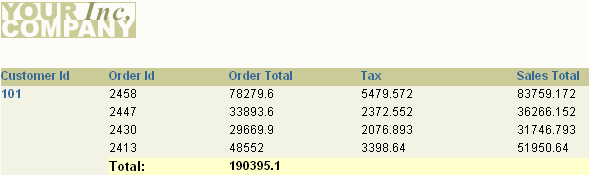# 14 Building a Group Left Formula Report

In this chapter, you will learn about group left formula reports, containing formula columns. By following the steps in this chapter, you can generate the report output shown in Figure 14-1.

Figure 14-1 Formula report outputDescription of "Figure 14-1 Formula report output"

Concepts

• A formula column, like a summary column, is a computational column you create yourself. Unlike a summary, its values are calculated based on a PL/SQL formula you provide. The formula may use data from another column in the report, but is not required to do so.

• A formula column performs a user-defined computation on another column(s) data, including placeholder columns. Formula columns should not be used to set values for parameters.

• A formula column contains at least one column whose value or values are computed using a PL/SQL formula. Formula columns are similar in usage to summary columns.

Data Relationships

• To create a formula report, create a query and select your data. Next, create additional columns and add them to groups in your report. Specify their formulas in the column property sheets.

• As with summaries, you do not select formulas from the database. unlike summaries, which use packaged computations shipped with Oracle Reports, formula columns use formulas you provide by referencing PL/SQL functions. These formulas can be any legal PL/SQL constructs, which allow a great deal of flexibility in the formulas you use.

• A formula performs computations using data from a single record, which can span multiple columns. This is in contrast to a summary, which summarizes the data from multiple records in a single column.

Layout

This report uses the default group left format with no modifications.

Example Scenario

As you build this example report, you will:

To see a sample formula report, open the examples folder called `formula`, then open the Oracle Reports example report named `formula.rdf`. For details on how to open it, see "Accessing the Example Reports" in the Preface.